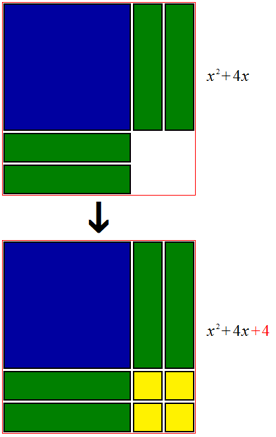38 PagesThis is one way of looking at completing the square, as represented by algebra tiles.

In algebra, completing the square is a mathematical method demonstrated by a man named al-Khwarzimi (780–850), who published a book that gave algebra its very name.

Suppose you were given the following mathematical expression: x2 + 16x. You have been asked to complete the square of this expression. How do you do that?

Here's how it's done:

1. Take the original expression...2. Factor out the x...3. Square the constant term (the term with no variable)...(or)

4. And apply it to the original expression. All squared terms remain positive when completing the square of an expression.Congratulations! You've completed the square!

## When There's a Coefficient

When coefficients are involved, the only major difference is the inclusion of one step: dividing the entire expression by that coefficient.

For instance, let's say you were given the following expression: 4x2 - 16x.

It's very easy, you know. All you have to do is divide the entire expression by 4.becomes.

Then, it's all downhill from here. Complete the square by following the steps above, and your answer should come up as this:## When the Linear Term Is An Odd Number

Suppose you were given the expression x2 + 7x. Here's what you do when the linear term is an odd number:

1. Factor out the x and put 7 over 2...

Fromto...

2. Square the fraction (there's an easy way)...3. And apply it to the expression. If you want to, you can simplify the improper fraction into a mixed number or decimal., which is alsoorThere you have it!

## Completing the Square to Solve Quadratic Equations

Al-Khwarzimi showed how to solve quadratic equations by finding the square by:

1. Rewriting the original problem...2. Moving the variables to different sides...3. Factoring the quadratic and linear terms to complete the square...4. Taking the square roots of both sides...5. And, finally, simplifying the whole thing.This is a little trickier, though it's easy once you get used to it.

## Now, Try This!

Complete the square to solve for x. Leave the answer in simplest radical form.Community content is available under CC-BY-SA unless otherwise noted.